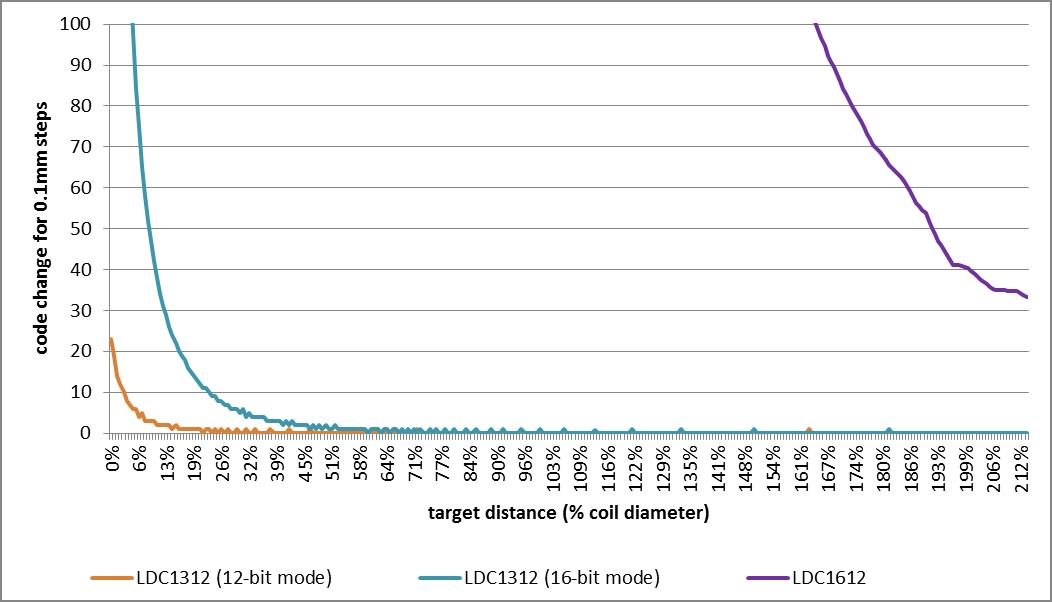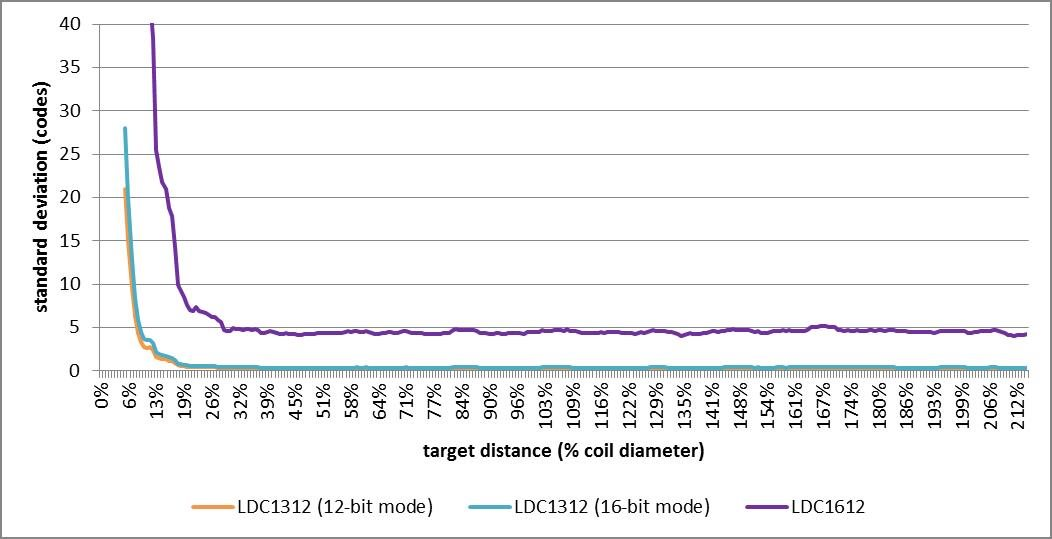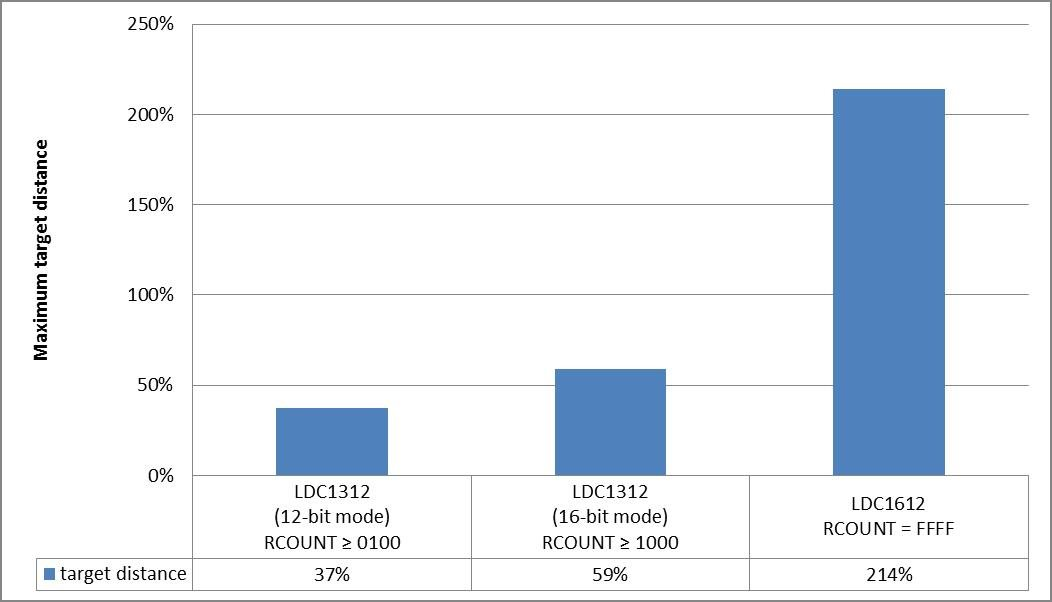# Inductive sensing: How far can I sense?

In my last few blog posts on inductive sensing, I introduced the latest addition to TI’s inductance-to-digital converter (LDC) portfolio: the multichannel LDC1612, LDC1614, LDC1312 and LDC1314. The LDC1312 and LDC1314 are 13.3KSPS 12-bit resolution converters, useful for a wide range of applications such as rotational knobs, keypads or flow meters. If system-boundary conditions allow, they can be configured in 16-bit mode without sacrificing the sampling rate. The dual-channel LDC1612 and quad-channel LDC1614 have integrated 28-bit data converters for use in very high-precision applications such as linear encoders or strain gauges.

How improved resolution increases sensing distance

The size of the magnetic field lines around a coil are proportional to the diameter of the sensor inductor. Therefore, the maximum sensing distance of the LDCs is a function of the coil diameter. The resolution and signal-to-noise ratio of the LDC do play a role, however, in determining how far a conductive object can be from the coil in order to detect its presence or measure its distance.

Using a 28-bit LDC over a 12-/16-bit LDC has two advantages:

• You can determine target position to higher accuracy.
• You can detect targets at longer distances.

Methodology and results

To determine the maximum target distance that the new multichannel LDCs can sense, I stepped an aluminum target in 0.1mm increments axially from the 14-mm diameter sensor coil of the LDC1612 evaluation module and captured the LDC response. I recorded both the code change between steps (Figure 1) and the standard deviation of 100 samples per step (Figure 2).Figure 1: Code change for 0.1mm steps versus target distanceFigure 2: Standard deviation versus target distance, 100 samples per step

Next, I used the measurement data on resolution and standard deviation to determine the maximum target sensing distance for the following conditions:

• The output code step size sufficient to resolve 0.1mm steps.
• The noise floor at the given target position is low enough to determine its position with 6σ probability (99.99966%).

By examining the data, I identified the distance above which these two conditions no longer hold true.This point is the maximum target distance that still meets my accuracy requirements above. The maximum target distance in each case is shown in Figure 3.Figure 3: Maximum target distance for resolving 0.1mm steps with 6σ probability

Guidelines for setting your target distance

The maximum target distance that you can sense varies with system parameters such as accuracy requirements and target material composition. Therefore, your application may have a longer or shorter maximum sensing range. However, since sensing range scales with coil diameter, it is possible to establish some rules of thumb from the data:

• LDCs such as the LDC1312 and LDC1314 operate best if the maximum target distance is kept within half of a coil diameter.
• In contrast, the high resolution of the channel LDC1612 and LDC1614 can be used to effectively sense targets as far as two coil diameters away from the sensor.

In my next post, I’ll explain how to configure the LDC1312 in 16-bit mode, which is beneficial in medium-resolution applications in which the faster sampling rates of the LDC1312 are preferable to the LDC1612.